5 You must Know things about today Reach me for Website Development, Mobile App Development, Digital Marketing and SEO services. Hidden things of Indian Politicians and Extreme research of Topics

# Torque on current loop in a uniform magnetic field

Torque on current loop in a uniform magnetic field:

As shown in fig. Consider a rectangular coil PQRS suspended in a uniform magnetic field , with its axis perpendicular to the field.

Let I = current flowing through the coil PQRS

a, b = sides of the coil PQRS

A = ab, area of the coil

θ = angle between the direction of B and that of the vector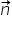drawn normal to the plane of the coil.

Now, a current carrying conductor experiences a force in a magnetic field.

therefore force on side PQ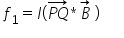Its magnitude is

f1 = laB sin(90-0) = IaB cosθ

therefore force on side QR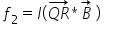Its magnitude is

f2 = lbB sin90 = IaB

therefore force on side RS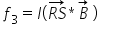Its magnitude is

F3 = laB sin(90+0) = IaB cosθ

therefore force on side SP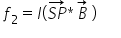Its magnitude is

F4 = lbB sin90 = IbB

The magnitude of the torque is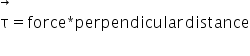= lbB * a sinθ = IBA sinθ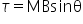Where m = lA = magnitude of the magnetic dipole moment.

In vector form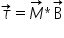In rectangular loop has N turns, the torque increases N times

τ=NBIAsinθ

Related Keywords